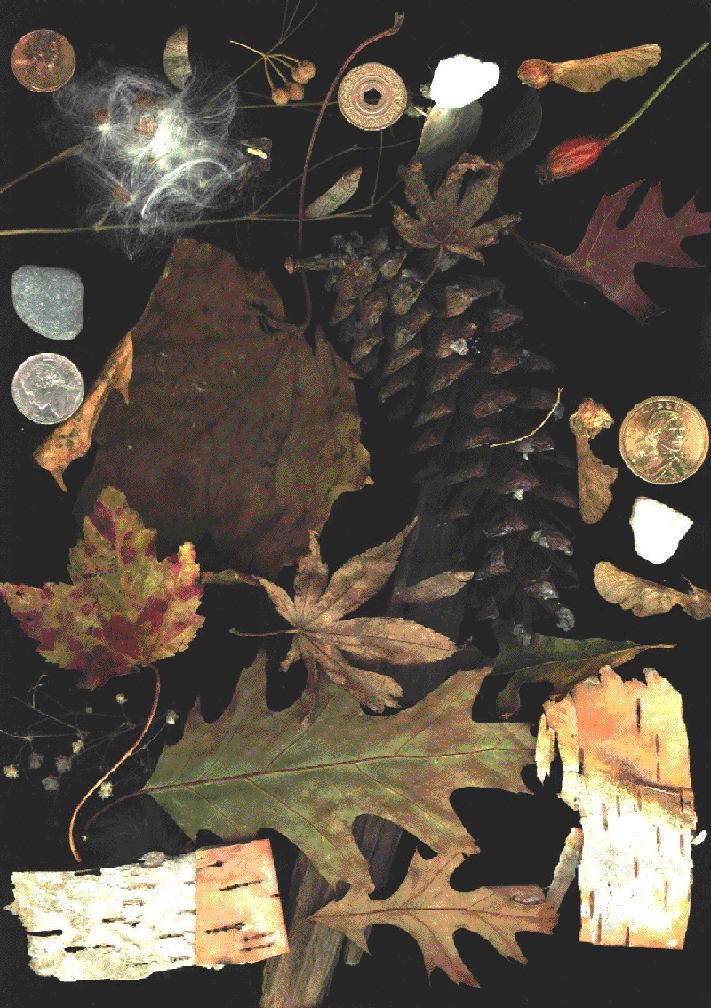HOME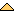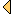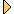P A 4     B  A  C  K   I  N   N  E  W  Y  O  R  K   A F T E R  T R I P  T O   P  L Y M  O T  H    M A S S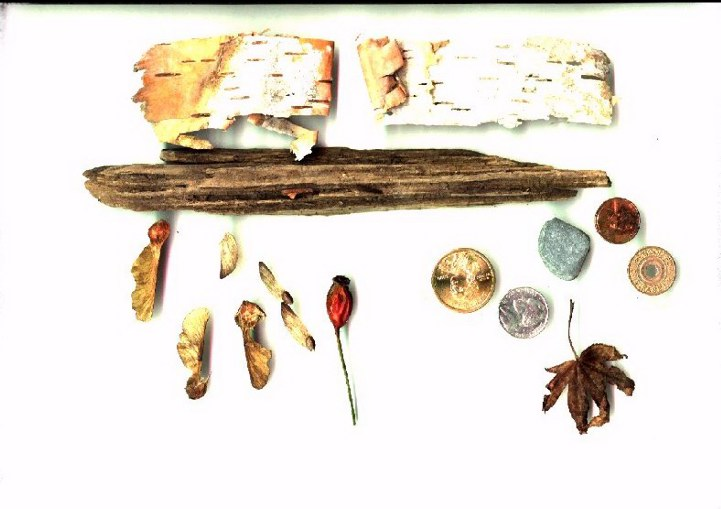B  A  C  K   I  N   N  E  W  Y  O  R  K   A F T E R  T R I P  T O   P  L Y M  O T  H    M A S S .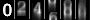© 1998-2001 Mingus Designs